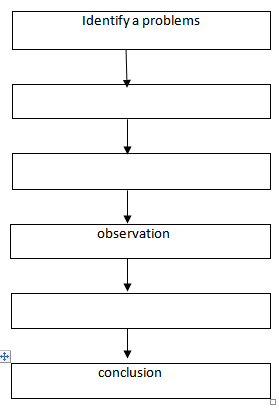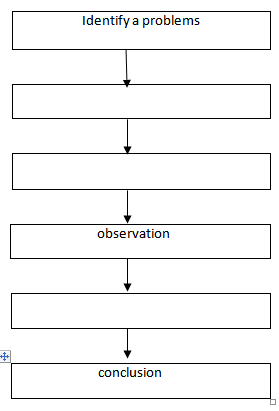CHEMISTRY O LEVEL(FORM ONE) – SCIENTIFIC PROCEDUREWritten by

SCIENTIFIC PROCEDURE

Chemistry is other science subject involves systematic ways of discovery, knowledge and solving problems. Discovering knowledge and solving problems involves very careful investigation.

IMPORTANCE OF SCIENTIFIC PROCEDURE

> Is the guide to the mental activities and systems needed for solving the competitiveness problems.

> To minimize the influence of bias or prejudice in the experiment.

> It provides standardized approach to conduct experiment and on doing so improves their result.

> It is an evidence based method for acquiring knowledge.

> It trains the brain to logically examine and process all the information it receivers.
These careful investigations involve facts and verifying them. Systematic investigation applied in chemistry includes the following steps: –
(i) Identify a problem
(ii) Hypothesis formulation
(iii) Experimentation
(iv) Observation
(v) Interpretation of data
(vi) Conclusion
(i) Identify a problem
This is the first step where a problem is recognized in a day life. One often comes across a question which requires explanation, such question is a problem needed to be answered by a chemist.
(ii) Hypothesis formulation
A hypothesis is a testable, assertion, statement or preposition about the relationship(s) between two or more phenomena. Hypothesis is an answer to the course of the problem that may be true or false. It is an intelligent guess to be possible answer to a problem. Sometime hypothesis can be started as an imagination that may lead either to confirmed truth or to biases about what is observed.
Hypothesis comes directly from the objectives. Hypothesis formulation is done after collecting all the available information and predicting an explanation of the phenomena. Any formulated hypothesis is never accepted unless it is proved through an experiment whether it is true or false.
(iii) Experimentation
An experiment is a practical activity which makes chemistry more realistic and interesting. It makes abstract concepts to be more understandable. Experiments are done to test hypothesizes where they are true or false.
After the experiment one may decide to present the data in an essay form, graphs, histograms, table or pie charts.

(iv) Observation
To see what is happening during the experiment and write or record the results.this may be done by using sense organ of eyes, nose, tongue, ears and skin. Recording all events that you consider relevant/important.
(v) Data interpretation
Data are the actual measurements that we make. Data is the plural form of datum. The data gathered are put into categories of particular trends in order to simplify their interpretation. The data analysis helps in drawing conclusion. A person analyzing the data should be capable of reading, interpreting and explaining any trends which it shows.

(vi) Conclusion
A hypothesis will be accepted /rejected on the results obtained from the experiment. When the results of the experiment come as it was predicted, then the hypothesis will be accepted as being correct. If the experimental results do not come out as expected the hypothesis will be rejected.

APPLICATION OF THE SCIENTIFIC PROCEDURE

The following are the common areas where the scientific procedures can be applied;

1. When carrying out experiment.

2. In project work; is a series of activities that allow the student to study, do research and act by themselves using their abilities , interests, personal experience and aptitudes.

3. In field study; is a collection of data that occurs outside of an experiment or laboratory setting.

REVIEW QUESTIONS
1. Define the following terms
(i) scientific procedure
(ii) hypothesis
(iii)experiment
(iv) conclusion
(v) observation
2. Fill in the boxes the missing step in the scientific procedure flow chart3. (a) Give the importance of scientific procedures.

(b) What are the application of scientific procedures.